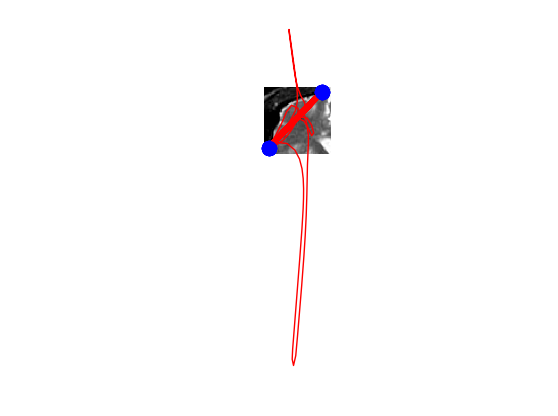# Active Contours using Parameteric Curves¶

This tour explores image segmentation using parametric active contours. $\newcommand{\dotp}{\langle #1, #2 \rangle}$ $\newcommand{\qandq}{\quad\text{and}\quad}$ $\newcommand{\qwhereq}{\quad\text{where}\quad}$ $\newcommand{\qifq}{ \quad \text{if} \quad }$ $\newcommand{\ZZ}{\mathbb{Z}}$ $\newcommand{\RR}{\mathbb{R}}$ $\newcommand{\CC}{\mathbb{C}}$ $\newcommand{\pa}{\left(#1\right)}$ $\newcommand{\si}{\sigma}$ $\newcommand{\Nn}{\mathcal{N}}$ $\newcommand{\Bb}{\mathcal{B}}$ $\newcommand{\EE}{\mathbb{E}}$ $\newcommand{\norm}{\|#1\|}$ $\newcommand{\abs}{\left|#1\right|}$ $\newcommand{\choice}{ \left\{ \begin{array}{l} #1 \end{array} \right. }$ $\newcommand{\al}{\alpha}$ $\newcommand{\la}{\lambda}$ $\newcommand{\ga}{\gamma}$ $\newcommand{\Ga}{\Gamma}$ $\newcommand{\La}{\Lambda}$ $\newcommand{\si}{\sigma}$ $\newcommand{\Si}{\Sigma}$ $\newcommand{\be}{\beta}$ $\newcommand{\de}{\delta}$ $\newcommand{\De}{\Delta}$ $\newcommand{\phi}{\varphi}$ $\newcommand{\th}{\theta}$ $\newcommand{\om}{\omega}$ $\newcommand{\Om}{\Omega}$

In :
using PyPlot
using NtToolBox
# arequire("NtToolBox")


## Parameteric Curves¶

In this tours, the active contours are represented using parametric curve $\ga : [0,1] \rightarrow \RR^2$.

This curve is discretized using a piewise linear curve with $p$ segments, and is stored as a complex vector of points in the plane $\ga \in \CC^p$.

Initial polygon.

In :
gamma0 = [.78, .14, .42, .18, .32, .16, .75, .83, .57, .68, .46, .40, .72, .79, .91, .90] + 1im*[.87, .82, .75, .63, .34, .17, .08, .46, .50, .25, .27, .57, .73, .57, .75, .79];


Display the initial curve.

In :
periodize = gamma -> [gamma; [gamma]]
function cplot(gamma, s = "b", lw = 1)
plot(real(periodize(gamma)), imag(periodize(gamma)), s, linewidth = lw)
axis("equal")
axis("off")
end
cplot(gamma0, "b.-")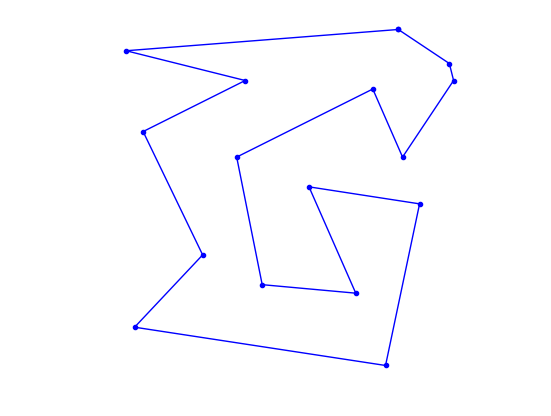Out:
(0.1015,0.9485,0.040499999999999994,0.9095)

Number of points of the discrete curve.

In :
p = 256;


Shortcut to re-sample a curve according to arc length.

In :
using Dierckx
curvabs = gamma -> [0; cumsum(1e-5 .+ abs(gamma[1:end - 1] - gamma[2:end]) )]
resample11 = (gamma, d) -> evaluate(Spline1D(d./d[end], real(gamma), k=1), (0:p-1)./p)
resample12 = (gamma, d) -> evaluate(Spline1D(d./d[end], imag(gamma), k=1), (0:p-1)./p)
resample = gamma -> resample11( [gamma; gamma], curvabs( [gamma; gamma] ) ) + 1im*resample12( [gamma; gamma], curvabs( [gamma; gamma] ) );

ArgumentError: Module Dierckx not found in current path.
Run Pkg.add("Dierckx") to install the Dierckx package.

in require(::Symbol) at /Applications/Julia-0.5.app/Contents/Resources/julia/lib/julia/sys.dylib:?

Initial curve $\ga_1(t)$.

In :
gamma1 = resample(gamma0);

Out:
256-element Array{Complex{Float64},1}:
0.78+0.87im
0.761553+0.868559im
0.743106+0.867118im
0.724659+0.865676im
0.706212+0.864235im
0.687764+0.862794im
0.669317+0.861353im
0.65087+0.859912im
0.632423+0.858471im
0.613976+0.857029im
0.595529+0.855588im
0.577082+0.854147im
0.558635+0.852706im
⋮
0.889715+0.719573im
0.899979+0.734968im
0.909894+0.750424im
0.905407+0.768371im
0.900921+0.786317im
0.887764+0.798157im
0.872369+0.808421im
0.856974+0.818684im
0.841579+0.828947im
0.826185+0.83921im
0.81079+0.849474im
0.795395+0.859737im

Display the initial curve.

In :
cplot(gamma1, "k");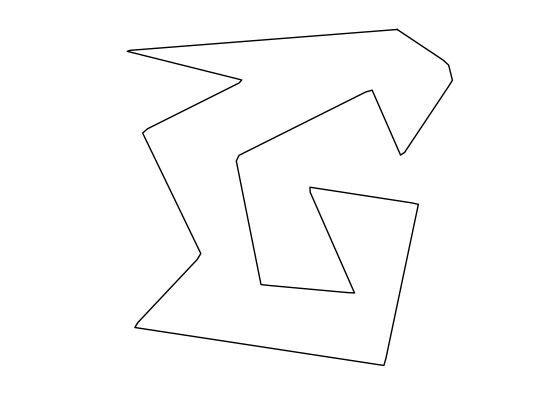Out:
(0.10727700934498144,0.9481138973125205,0.040705710689687556,0.909490204252872)

Shortcut for forward and backward finite differences.

In :
BwdDiff = c -> c - [c[end]; c[1:end - 1]]
FwdDiff = c -> [c[2:end]; c] - c
dotp = (c1, c2) -> real(c1.*conj(c2));

Out:
(::#15) (generic function with 1 method)

The tangent to the curve is computed as $$t_\ga(s) = \frac{\ga'(t)}{\norm{\ga'(t)}}$$ and the normal is $n_\ga(t) = t_\ga(t)^\bot.$

Shortcut to compute the tangent and the normal to a curve.

In :
normalize = v -> v./max(abs(v), 1e-10)
tangent = gamma -> normalize( FwdDiff(gamma) )
normal = gamma -> -1im*tangent(gamma);

Out:
(::#21) (generic function with 1 method)

Move the curve in the normal direction, by computing $\ga_1(t) \pm \delta n_{\ga_1}(t)$.

In :
delta = .03
gamma2 = gamma1 .+ delta .* normal(gamma1)
gamma3 = gamma1 .- delta .* normal(gamma1);

Out:
256-element Array{Complex{Float64},1}:
0.782337+0.840091im
0.76389+0.83865im
0.745442+0.837209im
0.726995+0.835768im
0.708548+0.834326im
0.690101+0.832885im
0.671654+0.831444im
0.653207+0.830003im
0.63476+0.828562im
0.616313+0.827121im
0.597866+0.825679im
0.579418+0.824238im
0.560971+0.822797im
⋮
0.864754+0.736214im
0.874728+0.751167im
0.88079+0.743148im
0.876303+0.761095im
0.880853+0.764018im
0.871123+0.773196im
0.855728+0.783459im
0.840333+0.793722im
0.824938+0.803986im
0.809544+0.814249im
0.794149+0.824512im
0.778754+0.834775im

Display the curves.

In :
cplot(gamma1, "k")
cplot(gamma2, "r--")
cplot(gamma3, "b--")
axis("tight")
axis("off")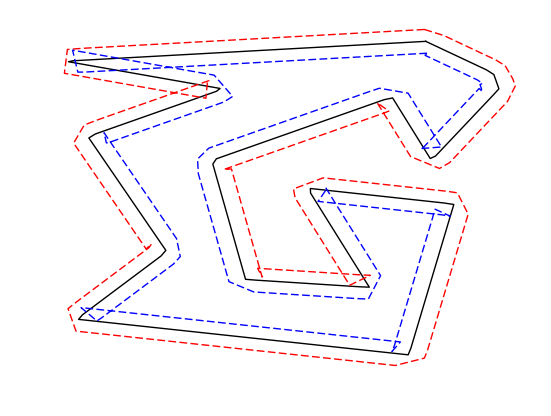Out:
(0.09818192340611892,0.979037189504653,0.011000256259768873,0.942237845572892)

## Evolution by Mean Curvature¶

A curve evolution is a series of curves $s \mapsto \ga_s$ indexed by an evolution parameter $s \geq 0$. The intial curve $\ga_0$ for $s=0$ is evolved, usually by minizing some energy $E(\ga)$ in a gradient descent $$\frac{\partial \ga_s}{\partial s} = \nabla E(\ga_s).$$

Note that the gradient of an energy is defined with respect to the curve-dependent inner product $$\dotp{a}{b} = \int_0^1 \dotp{a(t)}{b(t)} \norm{\ga'(t)} d t.$$ The set of curves can thus be thought as being a Riemannian surface.

The simplest evolution is the mean curvature evolution. It corresponds to minimization of the curve length $$E(\ga) = \int_0^1 \norm{\ga'(t)} d t$$

The gradient of the length is $$\nabla E(\ga)(t) = -\kappa_\ga(t) n_\ga(t)$$ where $\kappa_\ga$ is the curvature, defined as $$\kappa_\ga(t) = \frac{1}{\norm{\ga'(t)}} \dotp{ t_\ga'(t) }{ n_\ga(t) } .$$

Shortcut for normal times curvature $\kappa_\ga(t) n_\ga(t)$.

In :
normalC = gamma -> BwdDiff(tangent(gamma)) ./ abs( FwdDiff(gamma) );

Out:
(::#23) (generic function with 1 method)

Time step for the evolution. It should be very small because we use an explicit time stepping and the curve has strong curvature.

In :
dt = 0.001 / 100;

Out:
1.0e-5

Number of iterations.

In :
Tmax = 3.0 / 100
niter = round(Tmax/dt);

Out:
3000.0

Initialize the curve for $s=0$.

In :
gamma = gamma1;

Out:
256-element Array{Complex{Float64},1}:
0.78+0.87im
0.761553+0.868559im
0.743106+0.867118im
0.724659+0.865676im
0.706212+0.864235im
0.687764+0.862794im
0.669317+0.861353im
0.65087+0.859912im
0.632423+0.858471im
0.613976+0.857029im
0.595529+0.855588im
0.577082+0.854147im
0.558635+0.852706im
⋮
0.889715+0.719573im
0.899979+0.734968im
0.909894+0.750424im
0.905407+0.768371im
0.900921+0.786317im
0.887764+0.798157im
0.872369+0.808421im
0.856974+0.818684im
0.841579+0.828947im
0.826185+0.83921im
0.81079+0.849474im
0.795395+0.859737im

Evolution of the curve.

In :
gamma = gamma .+ dt .* normalC(gamma);

Out:
256-element Array{Complex{Float64},1}:
0.779911+0.869658im
0.761553+0.868559im
0.743106+0.867118im
0.724659+0.865676im
0.706212+0.864235im
0.687764+0.862794im
0.669317+0.861353im
0.65087+0.859912im
0.632423+0.858471im
0.613976+0.857029im
0.595529+0.855588im
0.577082+0.854147im
0.558635+0.852706im
⋮
0.889715+0.719573im
0.899971+0.734974im
0.909471+0.750493im
0.905407+0.768371im
0.900638+0.786147im
0.887716+0.798096im
0.872369+0.808421im
0.856974+0.818684im
0.841579+0.828947im
0.826185+0.83921im
0.81079+0.849474im
0.795395+0.859737im

To stabilize the evolution, it is important to re-sample the curve so that it is unit-speed parametrized. You do not need to do it every time step though (to speed up).

In :
gamma = resample(gamma);

Out:
256-element Array{Complex{Float64},1}:
0.779911+0.869658im
0.76173+0.868569im
0.743572+0.867154im
0.725414+0.865735im
0.707255+0.864317im
0.689097+0.862898im
0.670939+0.86148im
0.652781+0.860061im
0.634623+0.858642im
0.616465+0.857224im
0.598307+0.855805im
0.580149+0.854387im
0.56199+0.852968im
⋮
0.890755+0.721134im
0.900799+0.736326im
0.909116+0.752056im
0.905023+0.769802im
0.89969+0.787023im
0.886136+0.799159im
0.871021+0.80932im
0.855866+0.819423im
0.840712+0.829526im
0.825557+0.839629im
0.810403+0.849732im
0.795246+0.859832im

Exercise 1: Perform the curve evolution. You need to resample it a few times.

In :
include("NtSolutions/segmentation_2_snakes_param/exo1.jl")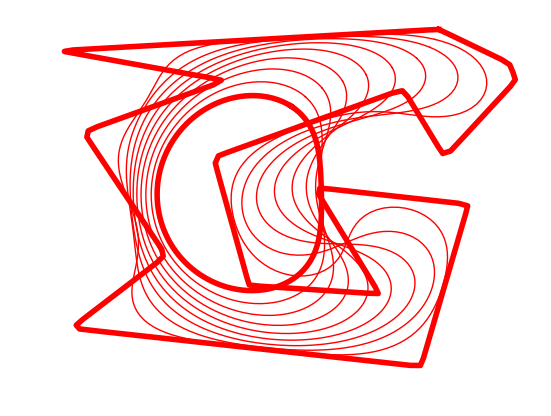## Geodesic Active Contours¶

Geodesic active contours minimize a weighted length $$E(\ga) = \int_0^1 W(\ga(t)) \norm{\ga'(t)} d t,$$ where $W(x)>0$ is the geodesic metric, that should be small in areas where the image should be segmented.

Size of the image $n$.

In :
n = 200;

Out:
200

Create a synthetic weight $W(x)$.

In :
nbumps = 40
theta = rand(nbumps,1).*(2*pi)
r = .6*n/2
a = [.62*n,.6*n]
x = round( a + r*cos(theta) )
y = round( a + r*sin(theta) )
W = zeros(n, n)
for i in 1:nbumps
W[Int(x[i]), Int(y[i])] = 1
end
W = gaussian_blur(W, 6.0)
W = rescale( -min(W, .05), .3,1);

Out:
200×200 Array{Float64,2}:
1.0  1.0  1.0  1.0  1.0  1.0  1.0  1.0  …  1.0  1.0  1.0  1.0  1.0  1.0  1.0
1.0  1.0  1.0  1.0  1.0  1.0  1.0  1.0     1.0  1.0  1.0  1.0  1.0  1.0  1.0
1.0  1.0  1.0  1.0  1.0  1.0  1.0  1.0     1.0  1.0  1.0  1.0  1.0  1.0  1.0
1.0  1.0  1.0  1.0  1.0  1.0  1.0  1.0     1.0  1.0  1.0  1.0  1.0  1.0  1.0
1.0  1.0  1.0  1.0  1.0  1.0  1.0  1.0     1.0  1.0  1.0  1.0  1.0  1.0  1.0
1.0  1.0  1.0  1.0  1.0  1.0  1.0  1.0  …  1.0  1.0  1.0  1.0  1.0  1.0  1.0
1.0  1.0  1.0  1.0  1.0  1.0  1.0  1.0     1.0  1.0  1.0  1.0  1.0  1.0  1.0
1.0  1.0  1.0  1.0  1.0  1.0  1.0  1.0     1.0  1.0  1.0  1.0  1.0  1.0  1.0
1.0  1.0  1.0  1.0  1.0  1.0  1.0  1.0     1.0  1.0  1.0  1.0  1.0  1.0  1.0
1.0  1.0  1.0  1.0  1.0  1.0  1.0  1.0     1.0  1.0  1.0  1.0  1.0  1.0  1.0
1.0  1.0  1.0  1.0  1.0  1.0  1.0  1.0  …  1.0  1.0  1.0  1.0  1.0  1.0  1.0
1.0  1.0  1.0  1.0  1.0  1.0  1.0  1.0     1.0  1.0  1.0  1.0  1.0  1.0  1.0
1.0  1.0  1.0  1.0  1.0  1.0  1.0  1.0     1.0  1.0  1.0  1.0  1.0  1.0  1.0
⋮                        ⋮              ⋱            ⋮
1.0  1.0  1.0  1.0  1.0  1.0  1.0  1.0     1.0  1.0  1.0  1.0  1.0  1.0  1.0
1.0  1.0  1.0  1.0  1.0  1.0  1.0  1.0     1.0  1.0  1.0  1.0  1.0  1.0  1.0
1.0  1.0  1.0  1.0  1.0  1.0  1.0  1.0  …  1.0  1.0  1.0  1.0  1.0  1.0  1.0
1.0  1.0  1.0  1.0  1.0  1.0  1.0  1.0     1.0  1.0  1.0  1.0  1.0  1.0  1.0
1.0  1.0  1.0  1.0  1.0  1.0  1.0  1.0     1.0  1.0  1.0  1.0  1.0  1.0  1.0
1.0  1.0  1.0  1.0  1.0  1.0  1.0  1.0     1.0  1.0  1.0  1.0  1.0  1.0  1.0
1.0  1.0  1.0  1.0  1.0  1.0  1.0  1.0     1.0  1.0  1.0  1.0  1.0  1.0  1.0
1.0  1.0  1.0  1.0  1.0  1.0  1.0  1.0  …  1.0  1.0  1.0  1.0  1.0  1.0  1.0
1.0  1.0  1.0  1.0  1.0  1.0  1.0  1.0     1.0  1.0  1.0  1.0  1.0  1.0  1.0
1.0  1.0  1.0  1.0  1.0  1.0  1.0  1.0     1.0  1.0  1.0  1.0  1.0  1.0  1.0
1.0  1.0  1.0  1.0  1.0  1.0  1.0  1.0     1.0  1.0  1.0  1.0  1.0  1.0  1.0
1.0  1.0  1.0  1.0  1.0  1.0  1.0  1.0     1.0  1.0  1.0  1.0  1.0  1.0  1.0

Display the metric $W$.

In :
imageplot(W)Pre-compute the gradient $\nabla W(x)$ of the metric.

In :
G = grad(W)
G = G[:,:,1] .+ 1im*G[:, :, 2];

Out:
200×200 Array{Complex{Float64},2}:
0.0+0.0im  …                   0.0+0.0im
0.0+0.0im                      0.0+0.0im
0.0+0.0im                      0.0+0.0im
0.0+0.0im                      0.0+0.0im
0.0+0.0im                      0.0+0.0im
0.0+0.0im  …                   0.0+0.0im
0.0+0.0im                      0.0+0.0im
0.0+0.0im                      0.0+0.0im
0.0+0.0im                      0.0+0.0im
0.0+0.0im                      0.0+0.0im
0.0+0.0im  …                   0.0+0.0im
0.0+0.0im                      0.0+0.0im
0.0+0.0im                      0.0+0.0im
⋮       ⋱
5.73319e-13+6.05738e-13im      1.39155e-12+1.44751e-12im
2.52687e-13+2.62457e-13im      6.15064e-13+6.29274e-13im
1.0858e-13+1.11022e-13im   …  2.64899e-13+2.66898e-13im
4.57412e-14+4.59632e-14im      1.11466e-13+1.10578e-13im
1.84297e-14+1.82077e-14im       4.55191e-14+4.4853e-14im
7.32747e-15+7.32747e-15im      1.82077e-14+1.77636e-14im
2.88658e-15+2.88658e-15im      7.10543e-15+6.88338e-15im
1.11022e-15+1.11022e-15im   …  2.88658e-15+2.66454e-15im
4.44089e-16+4.44089e-16im      8.88178e-16+8.88178e-16im
2.22045e-16+2.22045e-16im      4.44089e-16+4.44089e-16im
0.0+0.0im     2.22045e-16+2.22045e-16im
0.0+0.0im                      0.0+0.0im

Display the image of the magnitude $\norm{\nabla W(x)}$ of the gradient.

In :
imageplot(abs(G))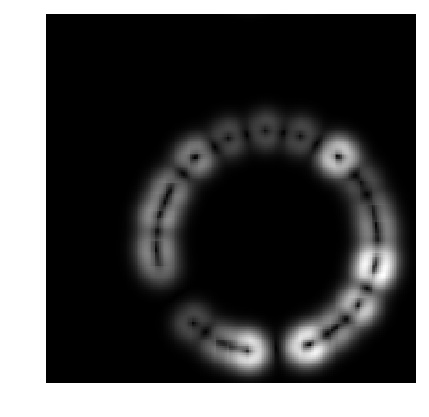Shortcut to evaluate the gradient and the potential along a curve.

In :
EvalG = gamma -> NtToolBox.bilinear_interpolate(G, imag(gamma), real(gamma))
EvalW = gamma -> NtToolBox.bilinear_interpolate(W, imag(gamma), real(gamma));

Out:
(::#27) (generic function with 1 method)

Create a circular curve $\ga_0$.

In :
r = .98*n/2 # radius
p = 128 # number of points on the curve
theta = transpose( linspace(0, 2*pi, p + 1) )
theta = theta[1 : end - 1]
gamma0 = n/2 * (1 + 1im) +  r*(cos(theta) + 1im*sin(theta));


Initialize the curve at time $t=0$ with a circle.

In :
gamma = gamma0;


For this experiment, the time step should be larger, because the curve is in $[0,n-1] \times [0,n-1]$.

In :
dt = 1;


Number of iterations.

In :
Tmax = 5000
niter = round(Tmax/ dt);


Display the curve on the background.

In :
lw = 2
clf
imageplot(transpose(W))
cplot(gamma, "r", lw)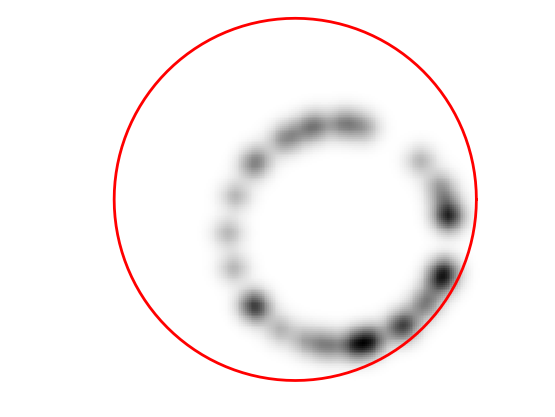Out:
(-0.5,199.5,199.5,-0.5)

The gradient of the energy is $$\nabla E(\ga) = -W(\ga(t)) \kappa_\ga(t) n_\ga(t) + \dotp{\nabla W(\ga(t))}{ n_\ga(t) } n_\ga(t).$$

Pointwise innerproduct on the curve.

In :
dotp = (c1,c2) -> real(c1.*conj(c2));

Out:
(::#29) (generic function with 1 method)

Evolution of the curve according to this gradient.

In :
N = normal(gamma)
g = - EvalW(gamma) .* normalC(gamma) + dotp(EvalG(gamma), N) .* N
gamma = (gamma - dt*g);

Out:
128-element Array{Complex{Float64},1}:
197.99+100.0im
197.872+104.808im
197.518+109.605im
196.929+114.378im
196.107+119.117im
195.053+123.81im
193.77+128.445im
192.262+133.012im
190.531+137.499im
188.582+141.896im
186.419+146.192im
184.049+150.377im
181.475+154.44im
⋮
181.476+45.5598im
184.049+49.6232im
186.419+53.8079im
188.582+58.104im
190.531+62.5009im
192.262+66.9882im
193.77+71.5551im
195.053+76.1904im
196.107+80.8831im
196.929+85.6219im
197.518+90.3953im
197.872+95.1919im

To avoid the curve from being poorly sampled, it is important to re-sample it evenly.

In :
gamma = resample(gamma);

Out:
128-element Array{Complex{Float64},1}:
197.99+100.0im
197.872+104.808im
197.518+109.605im
196.929+114.378im
196.107+119.117im
195.053+123.81im
193.77+128.445im
192.262+133.012im
190.531+137.499im
188.582+141.896im
186.419+146.192im
184.049+150.377im
181.475+154.44im
⋮
181.476+45.5598im
184.049+49.6232im
186.419+53.8079im
188.582+58.104im
190.531+62.5009im
192.262+66.9882im
193.77+71.5551im
195.053+76.1904im
196.107+80.8831im
196.929+85.6219im
197.518+90.3953im
197.872+95.1919im

Exercise 2: Perform the curve evolution.

In :
include("NtSolutions/segmentation_2_snakes_param/exo2.jl")

# gamma = copy(gamma0);
# displist = round(linspace(0, niter, 10))
# k = 1;
# clf;
# imageplot(transpose(W));
# track  = gamma
# for i in 0 : niter
#     N = normal(gamma);
#     g = EvalW(gamma) .* normalC(gamma) - dotp(EvalG(gamma), N) .* N;
#     gamma = resample( (gamma + dt*g));
#     if i == displist[k]
#         lw = 1
#         if (i == 0) | (i == niter)
#             lw = 4
#         end
#         cplot(gamma, "r", lw);
#         k = k + 1;
#         axis("equal"); axis("off");
#     end
# end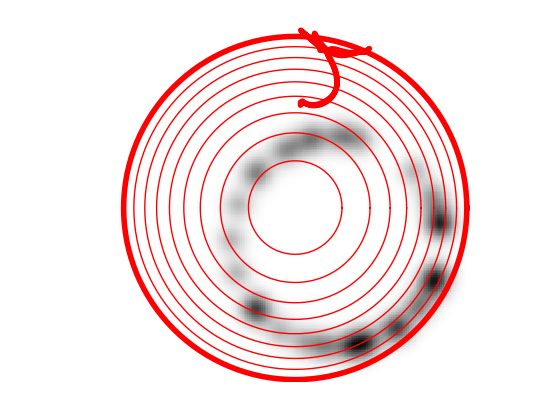# Medical Image Segmentation¶

One can use a gradient-based metric to perform edge detection in medical images.

Load an image $f$.

In :
n = 256
name = "NtToolBox/src/data/cortex.png"

Out:
256×256 Array{Float32,2}:
0.0  0.0  0.0  0.0  0.0  0.0  0.0  0.0  …  0.0  0.0  0.0  0.0  0.0  0.0  0.0
0.0  0.0  0.0  0.0  0.0  0.0  0.0  0.0     0.0  0.0  0.0  0.0  0.0  0.0  0.0
0.0  0.0  0.0  0.0  0.0  0.0  0.0  0.0     0.0  0.0  0.0  0.0  0.0  0.0  0.0
0.0  0.0  0.0  0.0  0.0  0.0  0.0  0.0     0.0  0.0  0.0  0.0  0.0  0.0  0.0
0.0  0.0  0.0  0.0  0.0  0.0  0.0  0.0     0.0  0.0  0.0  0.0  0.0  0.0  0.0
0.0  0.0  0.0  0.0  0.0  0.0  0.0  0.0  …  0.0  0.0  0.0  0.0  0.0  0.0  0.0
0.0  0.0  0.0  0.0  0.0  0.0  0.0  0.0     0.0  0.0  0.0  0.0  0.0  0.0  0.0
0.0  0.0  0.0  0.0  0.0  0.0  0.0  0.0     0.0  0.0  0.0  0.0  0.0  0.0  0.0
0.0  0.0  0.0  0.0  0.0  0.0  0.0  0.0     0.0  0.0  0.0  0.0  0.0  0.0  0.0
0.0  0.0  0.0  0.0  0.0  0.0  0.0  0.0     0.0  0.0  0.0  0.0  0.0  0.0  0.0
0.0  0.0  0.0  0.0  0.0  0.0  0.0  0.0  …  0.0  0.0  0.0  0.0  0.0  0.0  0.0
0.0  0.0  0.0  0.0  0.0  0.0  0.0  0.0     0.0  0.0  0.0  0.0  0.0  0.0  0.0
0.0  0.0  0.0  0.0  0.0  0.0  0.0  0.0     0.0  0.0  0.0  0.0  0.0  0.0  0.0
⋮                        ⋮              ⋱       ⋮                        ⋮
0.0  0.0  0.0  0.0  0.0  0.0  0.0  0.0     0.0  0.0  0.0  0.0  0.0  0.0  0.0
0.0  0.0  0.0  0.0  0.0  0.0  0.0  0.0  …  0.0  0.0  0.0  0.0  0.0  0.0  0.0
0.0  0.0  0.0  0.0  0.0  0.0  0.0  0.0     0.0  0.0  0.0  0.0  0.0  0.0  0.0
0.0  0.0  0.0  0.0  0.0  0.0  0.0  0.0     0.0  0.0  0.0  0.0  0.0  0.0  0.0
0.0  0.0  0.0  0.0  0.0  0.0  0.0  0.0     0.0  0.0  0.0  0.0  0.0  0.0  0.0
0.0  0.0  0.0  0.0  0.0  0.0  0.0  0.0     0.0  0.0  0.0  0.0  0.0  0.0  0.0
0.0  0.0  0.0  0.0  0.0  0.0  0.0  0.0  …  0.0  0.0  0.0  0.0  0.0  0.0  0.0
0.0  0.0  0.0  0.0  0.0  0.0  0.0  0.0     0.0  0.0  0.0  0.0  0.0  0.0  0.0
0.0  0.0  0.0  0.0  0.0  0.0  0.0  0.0     0.0  0.0  0.0  0.0  0.0  0.0  0.0
0.0  0.0  0.0  0.0  0.0  0.0  0.0  0.0     0.0  0.0  0.0  0.0  0.0  0.0  0.0
0.0  0.0  0.0  0.0  0.0  0.0  0.0  0.0     0.0  0.0  0.0  0.0  0.0  0.0  0.0
0.0  0.0  0.0  0.0  0.0  0.0  0.0  0.0  …  0.0  0.0  0.0  0.0  0.0  0.0  0.0

Display.

In :
imageplot(f)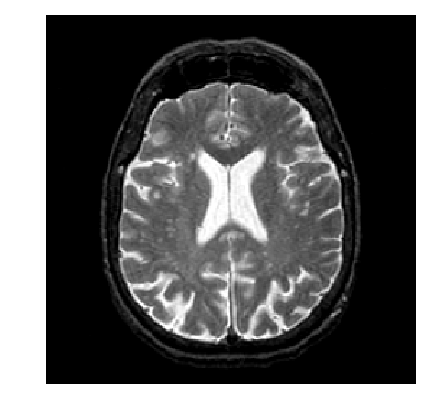An edge detector metric can be defined as a decreasing function of the gradient magnitude. $$W(x) = \psi( d \star h_a(x) ) \qwhereq d(x) = \norm{\nabla f(x)}.$$ where $h_a$ is a blurring kernel of width $a>0$.

Compute the magnitude of the gradient.

In :
G = grad(f)
d0 = sqrt(sum(G.^2, 3))
imageplot(d0[:, :, 1])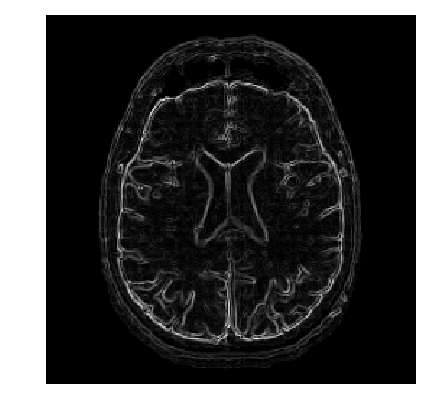Blur it by $h_a$.

In :
a = 2
d = gaussian_blur(d0, a)
imageplot(d[:, :, 1])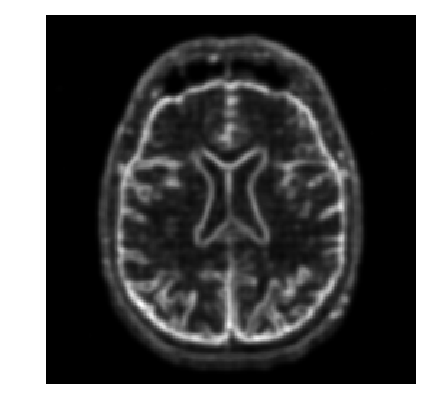In :
f = copy(d0)

n = maximum(size(f))
# t = [collect(0:n/2); collect(-n/2:-2)]
# (Y, X) = meshgrid(t, t)
# h = exp( -(X.^2 + Y.^2)./(2.0*float(sigma)^2) )
# h = h./sum(h)
# real(plan_ifft((plan_fft(f)*f).*(plan_fft(h)*h))*((plan_fft(f)*f).*(plan_fft(h)*h)))

Out:
128.0

Compute a decreasing function of the gradient to define $W$.

In :
d = min(d, .4)
W = rescale(-d, .8, 1);

Out:
256×256×1 Array{Float64,3}:
[:, :, 1] =
1.0  1.0  1.0  1.0  1.0  1.0  1.0  1.0  …  1.0  1.0  1.0  1.0  1.0  1.0  1.0
1.0  1.0  1.0  1.0  1.0  1.0  1.0  1.0     1.0  1.0  1.0  1.0  1.0  1.0  1.0
1.0  1.0  1.0  1.0  1.0  1.0  1.0  1.0     1.0  1.0  1.0  1.0  1.0  1.0  1.0
1.0  1.0  1.0  1.0  1.0  1.0  1.0  1.0     1.0  1.0  1.0  1.0  1.0  1.0  1.0
1.0  1.0  1.0  1.0  1.0  1.0  1.0  1.0     1.0  1.0  1.0  1.0  1.0  1.0  1.0
1.0  1.0  1.0  1.0  1.0  1.0  1.0  1.0  …  1.0  1.0  1.0  1.0  1.0  1.0  1.0
1.0  1.0  1.0  1.0  1.0  1.0  1.0  1.0     1.0  1.0  1.0  1.0  1.0  1.0  1.0
1.0  1.0  1.0  1.0  1.0  1.0  1.0  1.0     1.0  1.0  1.0  1.0  1.0  1.0  1.0
1.0  1.0  1.0  1.0  1.0  1.0  1.0  1.0     1.0  1.0  1.0  1.0  1.0  1.0  1.0
1.0  1.0  1.0  1.0  1.0  1.0  1.0  1.0     1.0  1.0  1.0  1.0  1.0  1.0  1.0
1.0  1.0  1.0  1.0  1.0  1.0  1.0  1.0  …  1.0  1.0  1.0  1.0  1.0  1.0  1.0
1.0  1.0  1.0  1.0  1.0  1.0  1.0  1.0     1.0  1.0  1.0  1.0  1.0  1.0  1.0
1.0  1.0  1.0  1.0  1.0  1.0  1.0  1.0     1.0  1.0  1.0  1.0  1.0  1.0  1.0
⋮                        ⋮              ⋱       ⋮                        ⋮
1.0  1.0  1.0  1.0  1.0  1.0  1.0  1.0     1.0  1.0  1.0  1.0  1.0  1.0  1.0
1.0  1.0  1.0  1.0  1.0  1.0  1.0  1.0  …  1.0  1.0  1.0  1.0  1.0  1.0  1.0
1.0  1.0  1.0  1.0  1.0  1.0  1.0  1.0     1.0  1.0  1.0  1.0  1.0  1.0  1.0
1.0  1.0  1.0  1.0  1.0  1.0  1.0  1.0     1.0  1.0  1.0  1.0  1.0  1.0  1.0
1.0  1.0  1.0  1.0  1.0  1.0  1.0  1.0     1.0  1.0  1.0  1.0  1.0  1.0  1.0
1.0  1.0  1.0  1.0  1.0  1.0  1.0  1.0     1.0  1.0  1.0  1.0  1.0  1.0  1.0
1.0  1.0  1.0  1.0  1.0  1.0  1.0  1.0  …  1.0  1.0  1.0  1.0  1.0  1.0  1.0
1.0  1.0  1.0  1.0  1.0  1.0  1.0  1.0     1.0  1.0  1.0  1.0  1.0  1.0  1.0
1.0  1.0  1.0  1.0  1.0  1.0  1.0  1.0     1.0  1.0  1.0  1.0  1.0  1.0  1.0
1.0  1.0  1.0  1.0  1.0  1.0  1.0  1.0     1.0  1.0  1.0  1.0  1.0  1.0  1.0
1.0  1.0  1.0  1.0  1.0  1.0  1.0  1.0     1.0  1.0  1.0  1.0  1.0  1.0  1.0
1.0  1.0  1.0  1.0  1.0  1.0  1.0  1.0  …  1.0  1.0  1.0  1.0  1.0  1.0  1.0

Display it.

In :
imageplot(W[:, :, 1])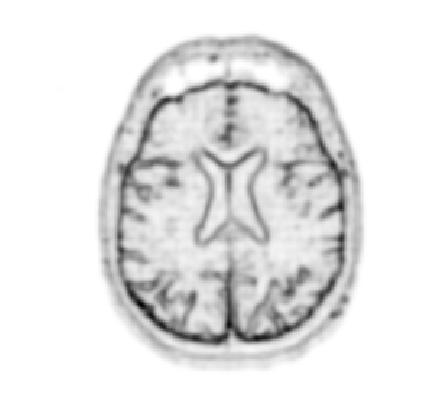Number of points.

In :
p = 128;


Exercise 3: Create an initial circle $\gamma_0$ of $p$ points. When plotting the image, you need to transpose it to have axis coherent with the cplot.

In :
include("NtSolutions/segmentation_2_snakes_param/exo3.jl")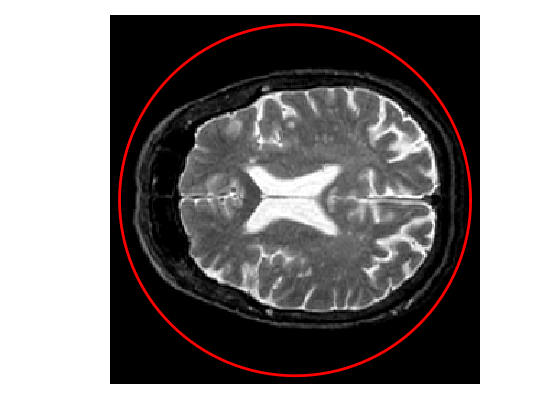Out:
(-0.5,255.5,255.5,-0.5)

Step size.

In :
dt = 2;


Number of iterations.

In :
Tmax = 9000
niter = round(Tmax/ dt);

Out:
4500.0

Exercise 4: Perform the curve evolution.

In :
include("NtSolutions/segmentation_2_snakes_param/exo4.jl")

# G = G[:, :, 1] + 1im*G[:, :, 2]
# EvalG = gamma -> NtToolBox.bilinear_interpolate(G, imag(gamma), real(gamma))
# EvalW = gamma -> NtToolBox.bilinear_interpolate(W, imag(gamma), real(gamma))
# #
# gamma = gamma0
# displist = round(linspace(0, niter, 10))
# k = 1;
# clf
# imageplot(transpose(f))
# for i in 0 : niter - 1
#     n = normal(gamma)
#     g = EvalW(gamma) .* normalC(gamma) - dotp(EvalG(gamma), n) .* n
#     gamma = resample( gamma + dt*g )
#     if i == displist[k]
#         lw = 1;
#         if (i == 0) | (i == niter)
#             lw = 4;
#         end
#         cplot(gamma, "r", lw);
#         k = k + 1;
#         axis("equal"); axis("off");
#     end
# end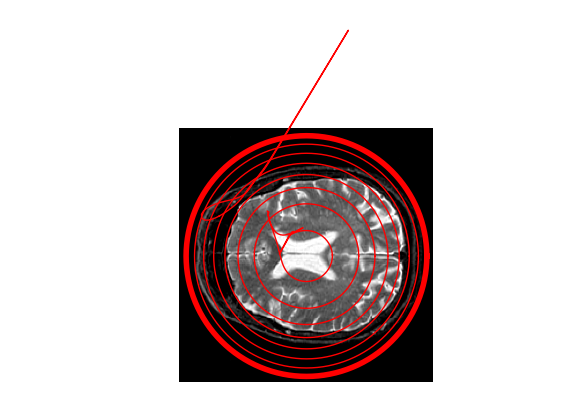# Evolution of a Non-closed Curve¶

It is possible to perform the evolution of a non-closed curve by adding boundary constraint $$\ga(0)=x_0 \qandq \ga(1)=x_1.$$

In this case, the algorithm find a local minimizer of the geodesic distance between the two points.

Note that a much more efficient way to solve this problem is to use the Fast Marching algorithm to find the global minimizer of the geodesic length.

Load an image $f$.

In :
n = 256
f = f[45:105, 60:120]
n = size(f);

Out:
61

Display.

In :
imageplot(f)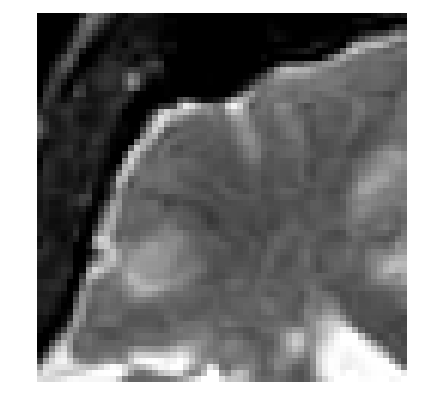Exercise 5: Compute an edge attracting criterion $W(x)>0$, that is small in area of strong gradient.

In :
include("NtSolutions/segmentation_2_snakes_param/exo5.jl")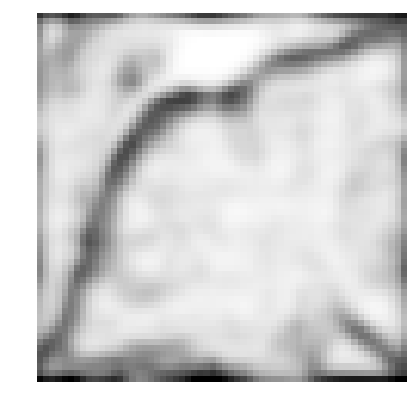Start and end points $x_0$ and $x_1$.

In :
x0 = 4 + 55*1im
x1 = 53 + 4*1im;

Out:
53 + 4im

Initial curve $\ga_0$.

In :
p = 128
t = transpose(linspace(0, 1, p))
gamma0 = t*x1 + (1 - t)*x0;

Out:
1×128 Array{Complex{Float64},2}:
4.0+55.0im  4.38583+54.5984im  …  52.6142+4.40157im  53.0+4.0im

Initialize the evolution.

In :
gamma = gamma0;

Out:
128-element Array{Complex{Float64},1}:
4.0+55.0im
4.38583+54.5984im
4.77165+54.1969im
5.15748+53.7953im
5.54331+53.3937im
5.92913+52.9921im
6.31496+52.5906im
6.70079+52.189im
7.08661+51.7874im
7.47244+51.3858im
7.85827+50.9843im
8.24409+50.5827im
8.62992+50.1811im
⋮
48.7559+8.41732im
49.1417+8.01575im
49.5276+7.61417im
49.9134+7.2126im
50.2992+6.81102im
50.685+6.40945im
51.0709+6.00787im
51.4567+5.6063im
51.8425+5.20472im
52.2283+4.80315im
52.6142+4.40157im
53.0+4.0im    

Display.

In :
clf
imageplot(transpose(W))
cplot(gamma[:],"r", 2)
plot(real(gamma), imag(gamma), "b.", markersize = 20)
plot(real(gamma[end]), imag(gamma[end]), "b.", markersize = 20);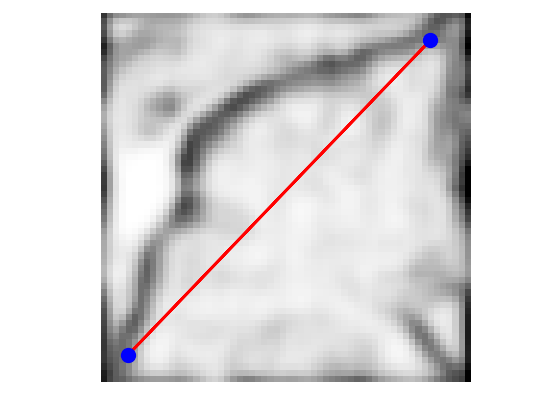Re-sampling for non-periodic curves.

In :
using Dierckx
curvabs = gamma -> [0; cumsum(1e-5 .+ abs(gamma[1 : end - 1] - gamma[2 : end]) )]
resample11 = (gamma, d) -> evaluate(Spline1D(d./d[end], real(gamma), k=1), (0:p-1)./p)
resample12 = (gamma, d) -> evaluate(Spline1D(d./d[end], imag(gamma), k=1), (0:p-1)./p)
resample = gamma -> resample11( [gamma; gamma], curvabs( [gamma; gamma] ) ) + 1im*resample12( [gamma; gamma], curvabs( [gamma; gamma] ) )

Out:
(::#57) (generic function with 1 method)

Time step.

In :
dt = 1/10;

Out:
0.1

Number of iterations.

In :
Tmax = 2000*4/ 7
niter = round(Tmax/ dt);

Out:
11429.0

Exercise 6: Perform the curve evolution. Be careful to impose the boundary conditions at each step.

In :
include("NtSolutions/segmentation_2_snakes_param/exo6.jl")